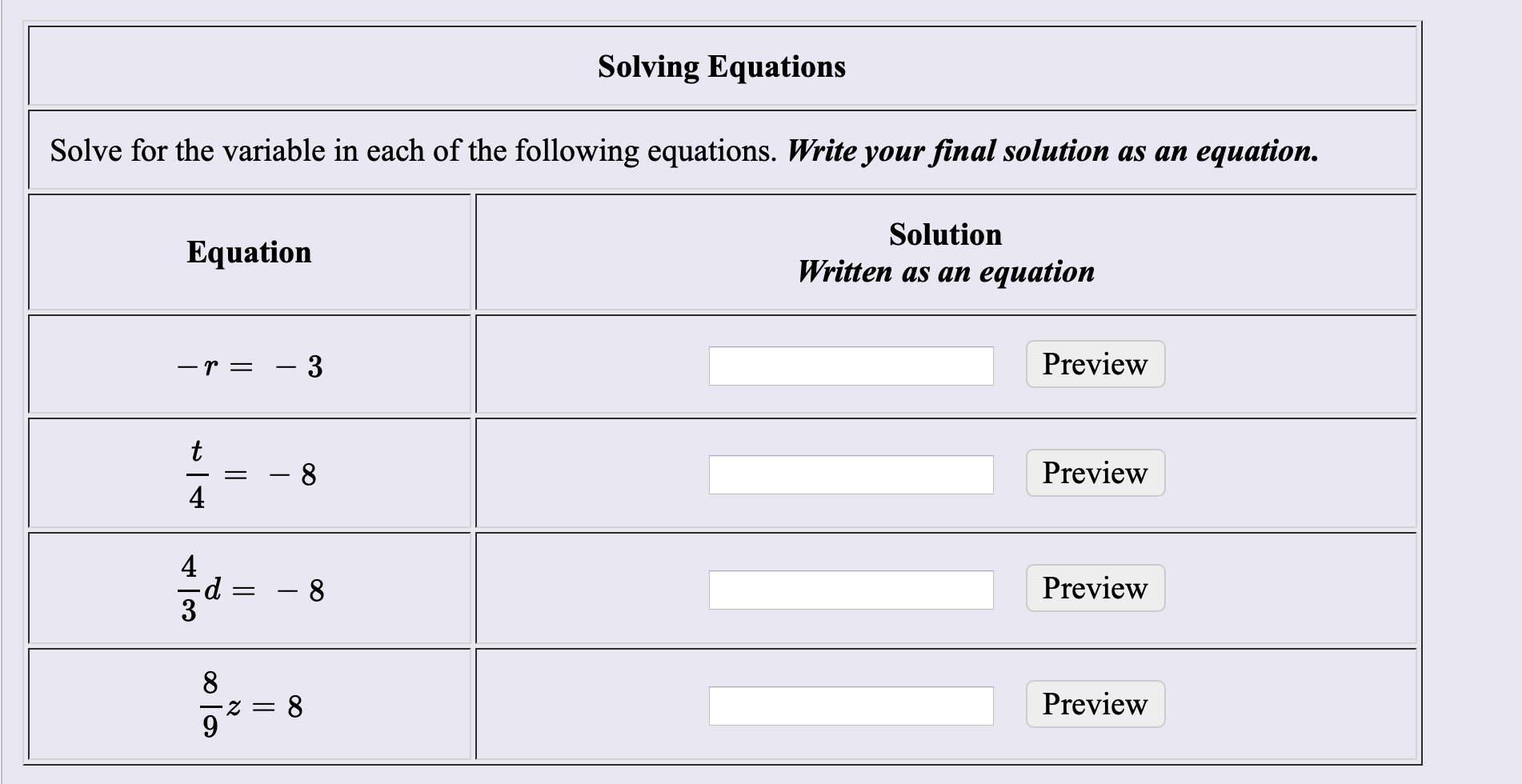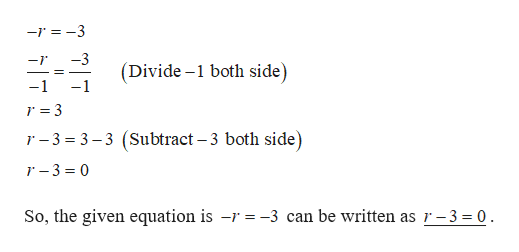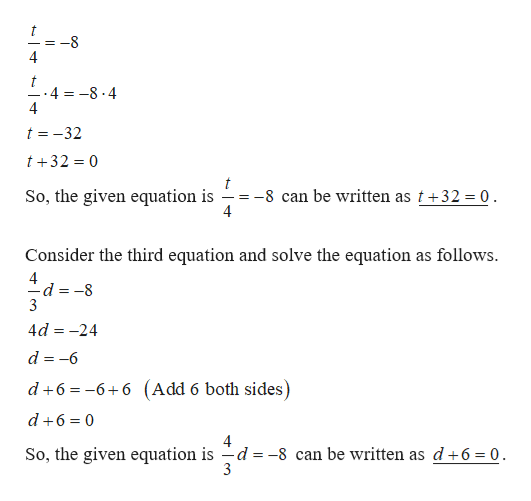# Solving EquationsSolve for the variable in each of the following equations. Write your final solution as anеquation.SolutionEquationWritten as anequationPreview- 3-r=tPreview844Preview8PreviewZ =со

Question
1 viewshelp_outlineImage TranscriptioncloseSolving Equations Solve for the variable in each of the following equations. Write your final solution as an еquation. Solution Equation Written as an equation Preview - 3 -r= t Preview 8 4 4 Preview 8 Preview Z = со fullscreen
check_circle

Step 1

Consider the first equation.

The given equation is –r = –3.

Solve the equation as follows.help_outlineImage Transcriptionclose-r-3 -3 -r (Divide -1 both side) -1 r = 3 r -3 3 3 (Subtract -3 both side r-3 0 So, the given equation is -r = -3 can be written as r-3 0 fullscreen
Step 2

Consider the second equation and So...help_outlineImage Transcriptioncloset 4 t 4 -8.4 4 t 32 t32 0 t -8 can be written as t+32 = 0 4 So, the given equation is _= as follows Consider the third equation and solve the equation -d =-8 3 4d 24 d -6 d+66+6 (Add 6 both sides d 6 0 So, the given equation is -d =-8 can be written as d+6 = 0. 3 fullscreen

### Want to see the full answer?

See Solution

#### Want to see this answer and more?

Solutions are written by subject experts who are available 24/7. Questions are typically answered within 1 hour.*

See Solution
*Response times may vary by subject and question.
Tagged in

### Equations and In-equations